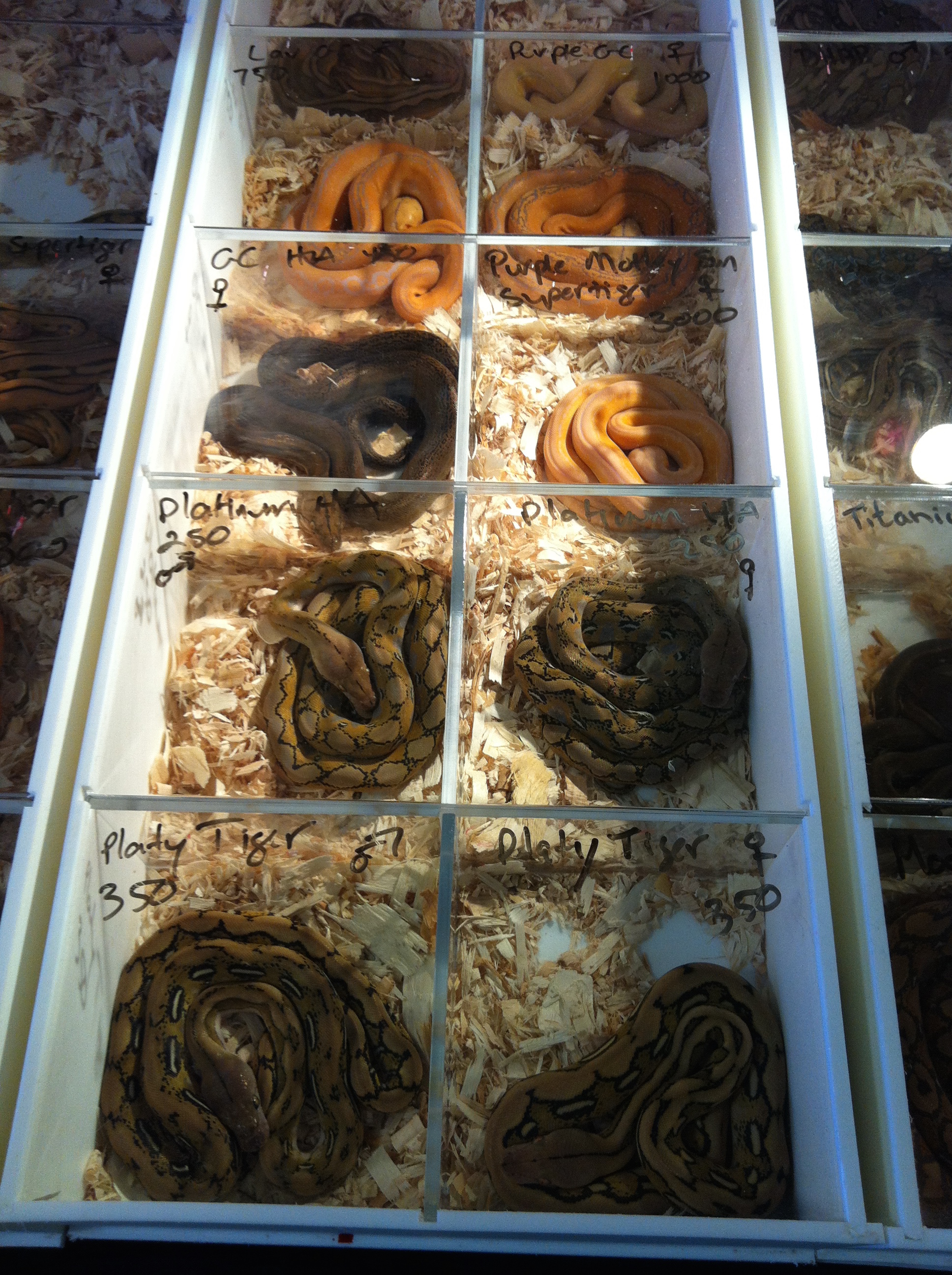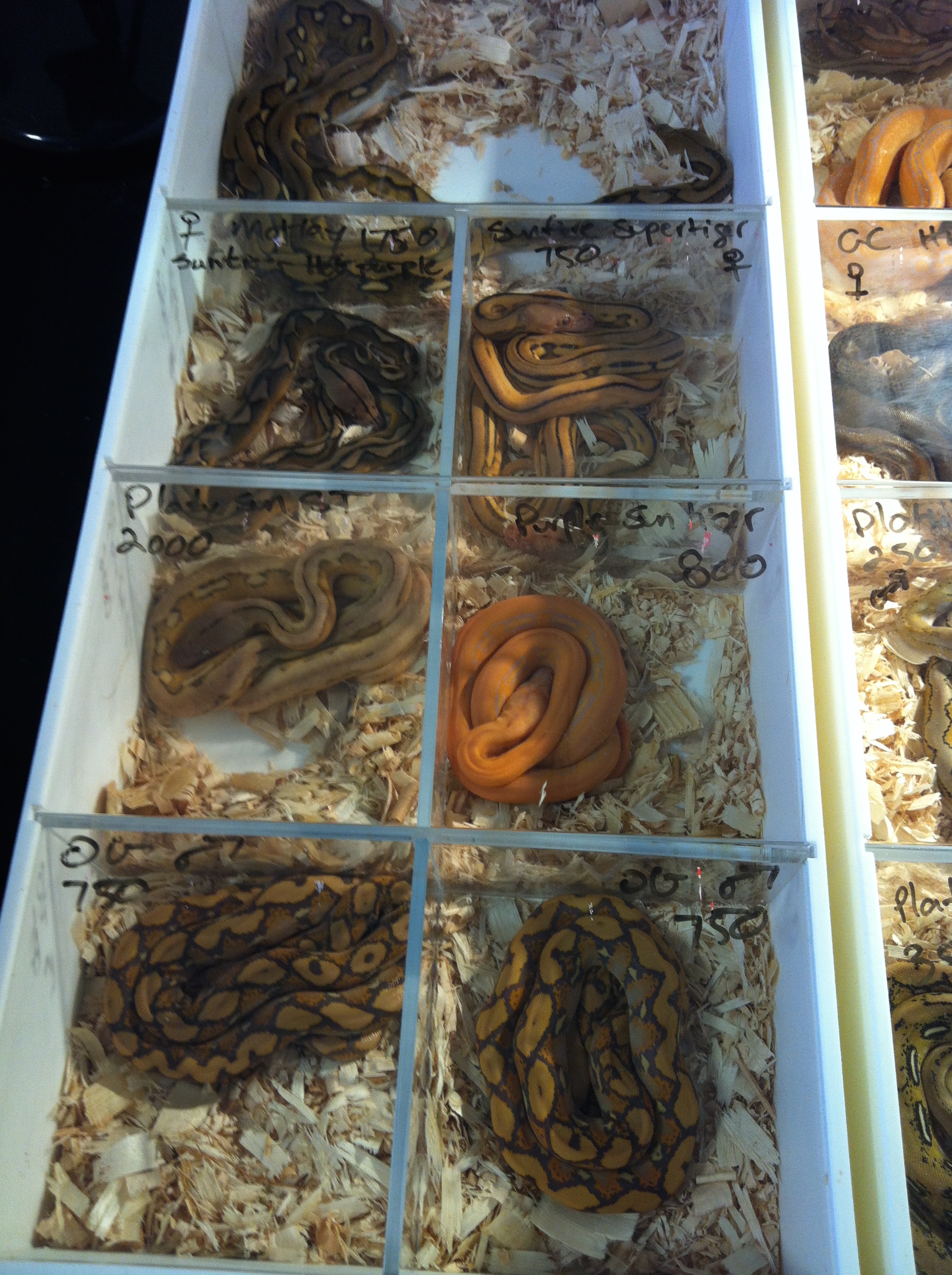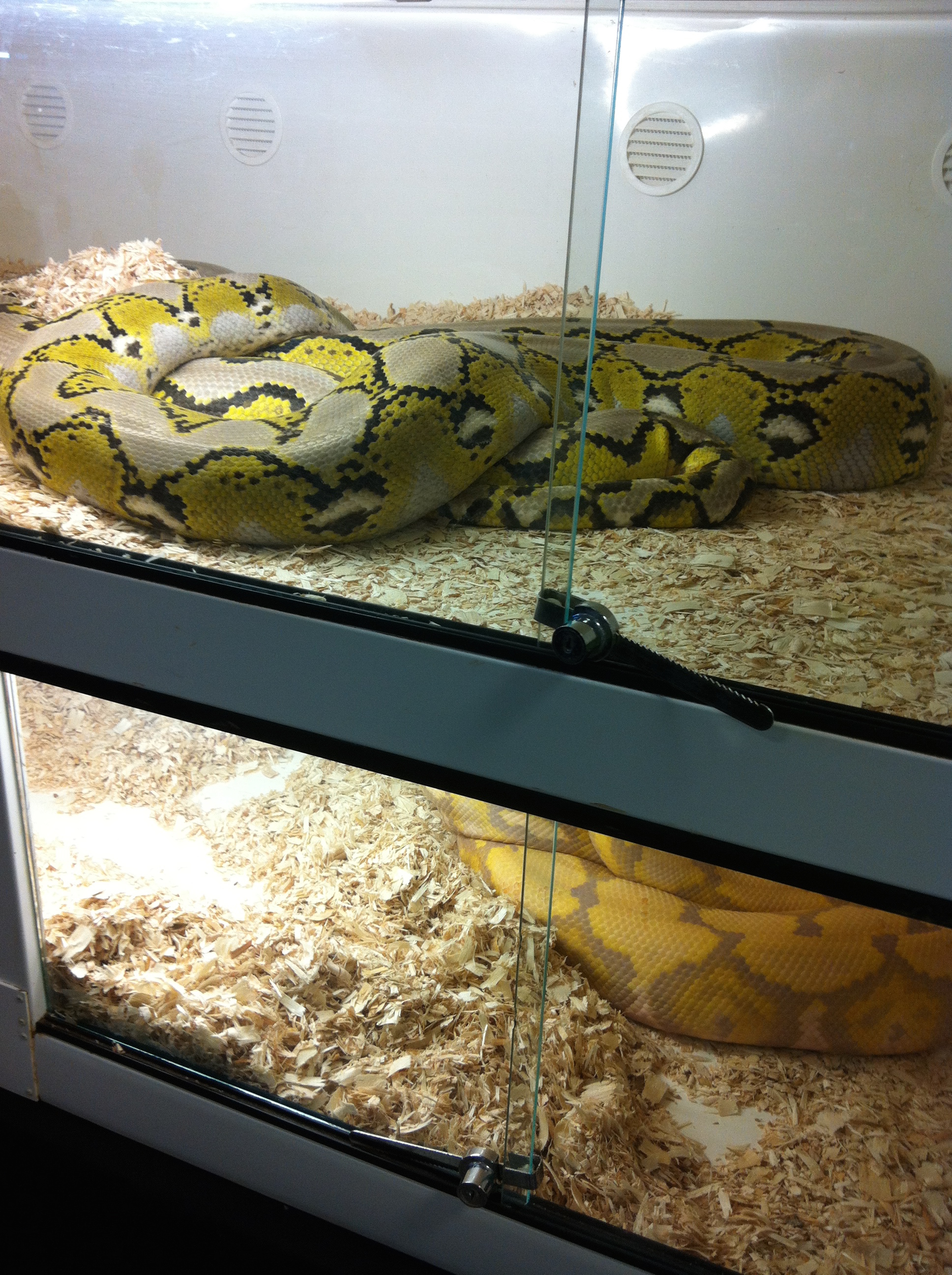∈ [1, 2)，发育成躯干；

∈ [2, 3)，发育成脑袋。

## 反应扩散模型 (reaction–diffusion system)

$$\frac{\partial u}{\partial t} = \underbrace{D_u \frac{\partial^2 u}{\partial^2 x}}_{Diffusion} + \overbrace{f(u)}^{Reaction}$$

## 趋化性模型 (chemotaxis model)

$$\begin{equation}\begin{split} rate \ of \ change \ of \ cell \ density&=diffusion &- chemotaxis + cell mitosis\\ \partial n / \partial t &= D_n \nabla^2 n &- \alpha \nabla \centerdot (n\nabla c) + rn(N - n)\\ rate \ of \ change \ of \ chemoattractant &= diffusion &+ chemoattractant \ production - degradation \\ \partial c / \partial t &= D_c \nabla^2 c &+ \frac{Sn}{\beta + n} - \gamma c \end{split}\end{equation}$$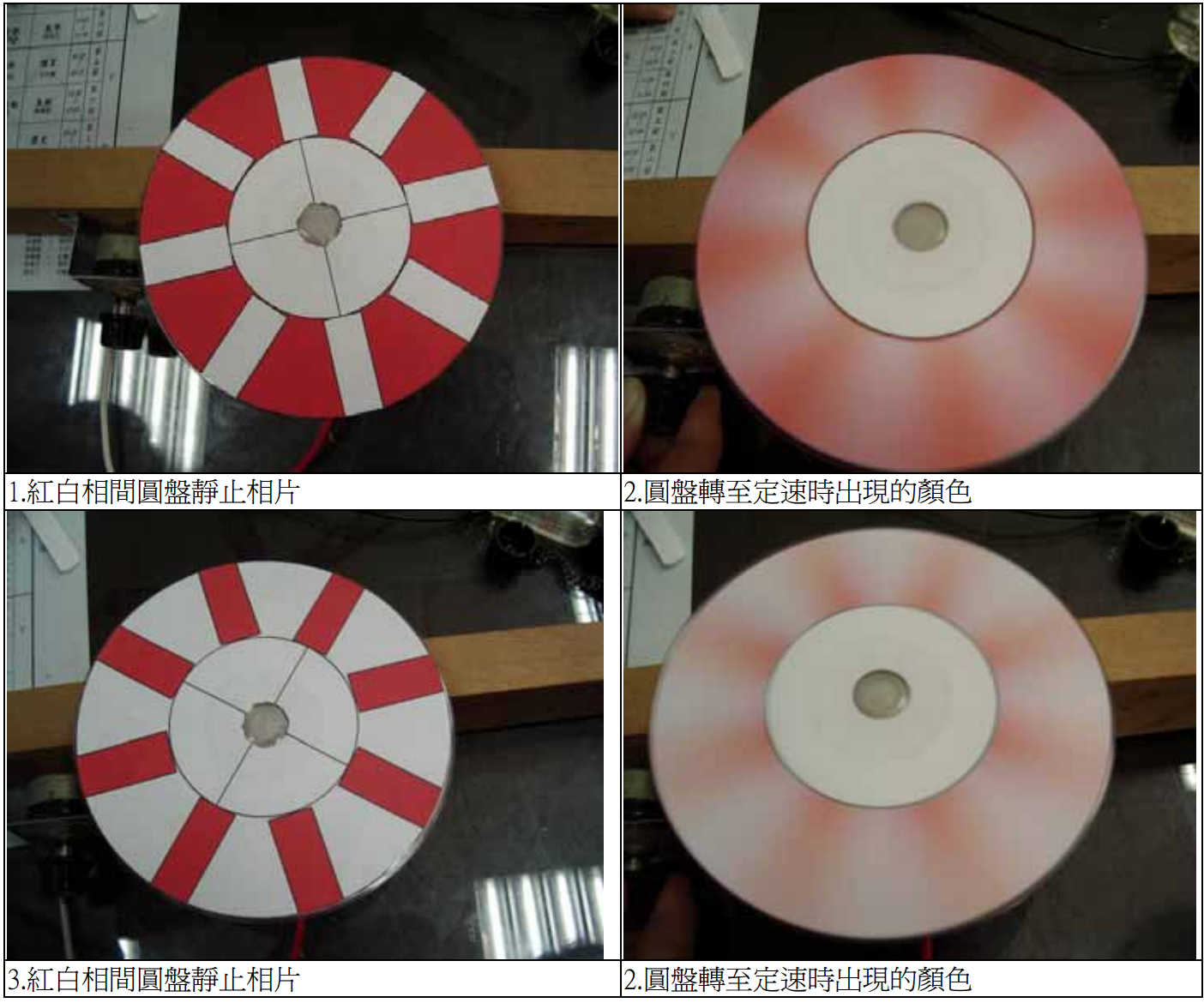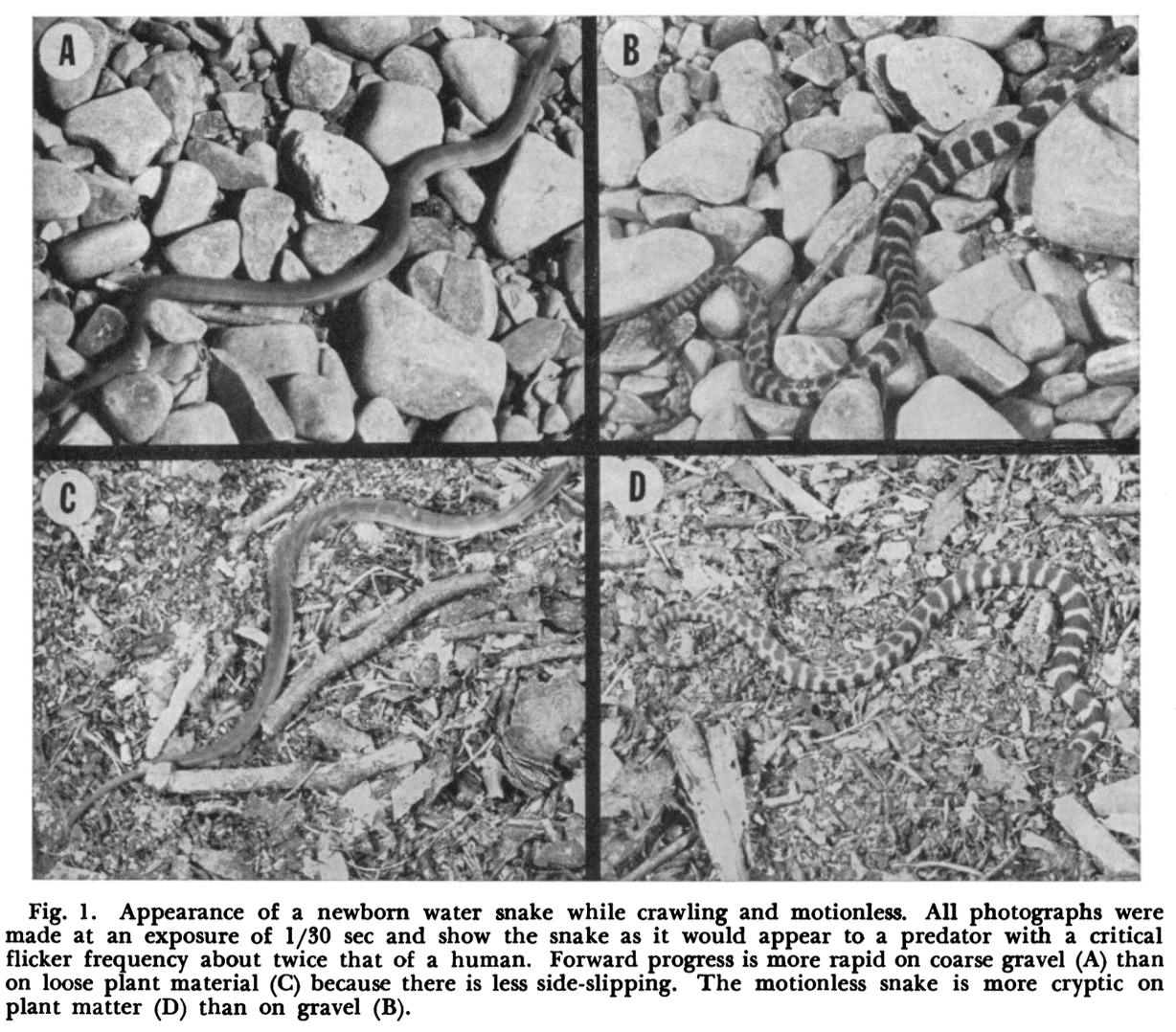右边是静止的蛇，左边是快速移动中的，在1/30秒的曝光中，看起来已经是单色的了。而一秒24帧在人眼看来就已经是连续的了。图片来源

1. 蛇的图样发育未必是以图灵模型所描述的方式形成的；

2. 即使是，该模型也并未禁止其他类型的图样，虽然形成其他图形的参数空间较小，但进化并不是一个随机过程；

3. 其实有很多的蛇是纵纹的，但它们大多是有毒的海蛇，而我去的是个爬宠卖展，那里卖的自然大部分是陆生无毒蛇，谁会搞个水生毒蛇当宠物啊。